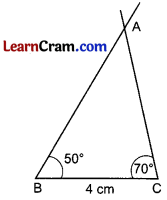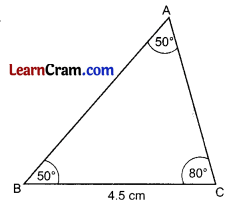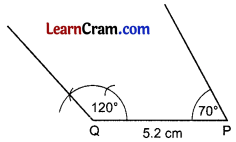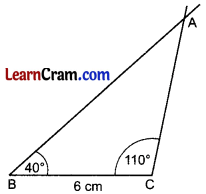# DAV Class 7 Maths Chapter 10 Worksheet 3 Solutions

The DAV Class 7 Maths Solution and DAV Class 7 Maths Chapter 10 Worksheet 3 Solutions of Construction of Triangles offer comprehensive answers to textbook questions.

## DAV Class 7 Maths Ch 10 WS 3 Solutions

Question 1.
Construct a ∆ABC in which BC = 4 cm, ∠B = 50° and ∠C = 70°
Steps of Construction:1. Draw BC = 4 cm.
2. Draw ∠B = 50° and ∠C = 70° to cut each other at A.
3. ∆ABC is the required triangle.

Question 2.
Draw a ∆ABC in which BC = 8 cm, ∠B = 50° and ∠A = 50°.
Steps of Construction:1. Draw BC = 8 cm.
2. Draw ∠B = 50° and ∠C = 80° to meet at A.Question 3.
Draw a triangle ABC with AB = 4.5 cm, ∠ABC = 35°, ∠ACB = 65°.
Steps of Construction:1. Draw AB = 4.5 cm. c
2. Draw ∠B = 35°
3. Draw ∠A = 80°
[∵ ∠A = 180° — (35° + 65°) = 80°]
4. They meet at C.
5. ∆ABC is the required triangle. A

Question 4.
Construct a ∆PQR with PQ = 7.5 cm, ∠RPQ = 45° ∠RQP = 45°. Measure the third angle. What kind of a triangle is it?
Steps of Construction:1. Draw PQ = 7.5 cm.
2. Draw an angle of 45° at P and at Q.
3. The two angle lines meet at R.
4. ∆PRQ is the required triangle.
5. ∠PRQ = 90°
6. ∆PQR is an isosceles right angled triangle.

Question 5.
Construct a ∆PQR with ∠P = 70°, ∠Q = 120° and side PQ = 5.2 cm. Is the construction possible?
Steps of Construction:1. Draw PQ = 5.2 cm
2. Draw ∠Q = 120° and ∠P = 70°
3. The two angle lines do not meet any point.
[∵ 120° + 70° > 180° ⇒ 190° > 180°]
4. Therefore, the construction of ∆PQR is not possible.Question 6.
Construct a ∆ABC in which BC